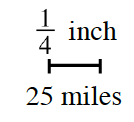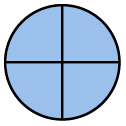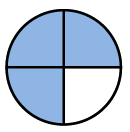### Home > CC2MN > Chapter 9 Unit 9 > Lesson CC2: 9.2.2 > Problem9-67

9-67.James lives in Columbus, OH, and wants to know how far he is from Indianapolis. On his map, the two cities are $1\frac{3}{4}$ inches apart. At the bottom of the map, he sees the scale at right. Write a proportion and then find the distance from Columbus to Indianapolis.  Homework Help ✎

If one fourth of an inch is equal to $25$ miles, you will need to find how many one fourths are in one and three fourths. Can you first find how many one fourths are in $1$?

James drew this diagram to help find how many one fourths are in $1$. Can you complete the diagram to find how many one fourths are in three fourths?The diagrams show that there are $7$ one fourths in one and three fourths. Use multiplication and the scale above to find how many miles there are from Columbus to Indianapolis.

James lives $175$ miles from Indianapolis. Did you remember to explain how you know?